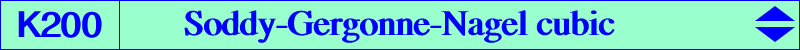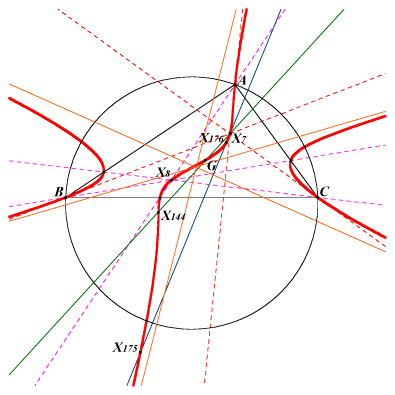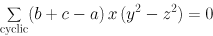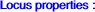other equations belowX(2), X(7), X(8), X(144), X(175), X(176), X(1143), X(1274)The Soddy-Gergonne-Nagel cubic K200 is pK(X2, X8). The pivot is the Nagel point X(8) and it passes through the Soddy centers X(175), X(176). See also K032 and K199. Its isogonal transform is pK(X32, X55). Its complement is K363. K170 and K200 meet at A, B, C, X(2), Ga, Gb, Gc and two other points with first barycentric coordinate sec(A) ± tan(A). These points are isotomic conjugates and lie on the line X(4), X(8). See ADGEOM, #3773 & sq., 2017-04-27.If A'B'C' is the cevian triangle of X then K200 is the locus of X for which BA' + CB' + AC' = A'C + B'A + C'B. For, if X = x:y:z, then it is required that : a z / (y + z) + b x / (z + x) + c y / (x + y) = a y / (y + z) + b z / (z + x) + c x / (x + y) from which we have : a (y - z)(z + x)(x + y) + b (z - x)(x + y)(y + z) + c (x - y)(y + z)(z + x) = 0 or equivalently : a (y - z) / (y + z) + cyclic = 0 (Richard Hilton, 2013-02-02). Compare with K007 and K034.